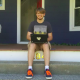author David ThompsonMon, 3 Sep 2018 02:12:27 +0000 (22:12 -0400) committer David ThompsonMon, 3 Sep 2018 02:21:49 +0000 (22:21 -0400)
* chickadee/math/grid.scm: New file.
* examples/grid.scm: New file.
* Makefile.am (SOURCES): Add grid module.
(EXTRA_DIST): Add grid example.

 Makefile.am patch | blob | blame | history chickadee/math/grid.scm [new file with mode: 0644] patch | blob examples/grid.scm [new file with mode: 0644] patch | blob

index 4640581..1ae2d24 100644 (file)
@@ -51,6 +51,7 @@ SOURCES =                                     \
@@ -83,6 +84,7 @@ EXTRA_DIST +=                                         \
examples/text.scm                            \
examples/nine-patch.scm                      \
examples/tiled.scm                           \
+  examples/grid.scm                            \
examples/images/AUTHORS                      \
examples/images/dialog-box.png               \
new file mode 100644 (file)
index 0000000..0361333
--- /dev/null
@@ -0,0 +1,404 @@
+;;; Chickadee Game Toolkit
+;;; Copyright © 2018 David Thompson <davet@gnu.org>
+;;;
+;;; Chickadee is free software: you can redistribute it and/or modify
+;;; it under the terms of the GNU General Public License as published
+;;; by the Free Software Foundation, either version 3 of the License,
+;;; or (at your option) any later version.
+;;;
+;;; Chickadee is distributed in the hope that it will be useful, but
+;;; WITHOUT ANY WARRANTY; without even the implied warranty of
+;;; MERCHANTABILITY or FITNESS FOR A PARTICULAR PURPOSE.  See the GNU
+;;; General Public License for more details.
+;;;
+;;; You should have received a copy of the GNU General Public License
+;;; along with this program.  If not, see
+
+;;; Commentary:
+;;
+;; Axis-aligned bounding box collision grid.
+;;
+;; Lots of inspiration drawn from https://github.com/kikito/bump.lua
+;;
+;;; Code:
+
+(define-module (chickadee math grid)
+  #:use-module (chickadee array-list)
+  #:use-module (chickadee math rect)
+  #:use-module (chickadee math vector)
+  #:use-module (ice-9 match)
+  #:use-module (srfi srfi-9)
+  #:use-module (srfi srfi-11)
+  #:export (slide
+            cell?
+            cell-count
+            make-grid
+            grid?
+            grid-cell-size
+            grid-remove
+            grid-move
+            grid-clear
+            grid-cell-count
+            grid-item-count
+            for-each-cell
+            for-each-item))
+
+\f
+;;;
+;;; Collision resolvers
+;;;
+
+(define (slide item item-rect other other-rect goal)
+  "Resolve the collision that occurs between ITEM and OTHER when
+moving ITEM-RECT to GOAL by sliding ITEM-RECT the minimum amount
+needed to make the rectangles no longer overlap."
+  (let* ((goal-x (vec2-x goal))
+         (goal-y (vec2-y goal))
+         (x1 (max goal-x (rect-left other-rect)))
+         (x2 (min (+ goal-x (rect-width item-rect)) (rect-right other-rect)))
+         (y1 (max goal-y (rect-bottom other-rect)))
+         (y2 (min (+ goal-y (rect-height item-rect)) (rect-top other-rect)))
+         (x-fix (- x2 x1))
+         (y-fix (- y2 y1)))
+    (if (< x-fix y-fix)
+        (if (= goal-x x1)
+            (set-vec2-x! goal (+ (vec2-x goal) x-fix))
+            (set-vec2-x! goal (+ (vec2-x goal) (- x-fix))))
+        (if (= goal-y y1)
+            (set-vec2-y! goal (+ (vec2-y goal) y-fix))
+            (set-vec2-y! goal (+ (vec2-y goal) (- y-fix)))))))
+
+\f
+;;;
+;;; Cells
+;;;
+
+(define-record-type <cell>
+  (make-cell items count)
+  cell?
+  (items cell-items)
+  (count cell-count set-cell-count!))
+
+(define (make-empty-cell)
+  (make-cell (make-hash-table) 0))
+
+(define (cell-add cell item)
+  (hashq-set! (cell-items cell) item #t)
+  (set-cell-count! cell (+ (cell-count cell) 1)))
+
+(define (cell-remove cell item)
+  (hashq-remove! (cell-items cell) item)
+  (set-cell-count! cell (- (cell-count cell) 1)))
+
+(define (for-each-cell-item proc cell)
+  "Call PROC with each item in CELL."
+  (hash-for-each (lambda (item unused)
+                   (proc item))
+                 (cell-items cell)))
+
+\f
+;;;
+;;; Grid
+;;;
+
+(define-record-type <grid>
+  (%make-grid cell-size rects rows scratch-rect buffer visited)
+  grid?
+  (cell-size grid-cell-size)
+  (rects grid-rects)
+  (rows grid-rows)
+  ;; The below fields are scratch space data structures that are
+  ;; allocated once when the grid is created to cut down on
+  ;; allocations while modifying the grid and checking for collisions.
+  (scratch-rect grid-scratch-rect)
+  (buffer grid-buffer)
+  (visited grid-visited))
+
+(define* (make-grid #:optional (cell-size 64))
+  "Create new grid partitioned by CELL-SIZE."
+  (%make-grid cell-size
+              (make-hash-table)
+              (make-hash-table)
+              (make-rect 0.0 0.0 0.0 0.0)
+              (make-array-list)
+              (make-hash-table)))
+
+(define (item-in-grid? grid item)
+  "Return #t if ITEM is in GRID."
+  (hashq-ref (grid-rects grid) item))
+
+(define (grid-row-ref grid y)
+  "Return the row at index Y in GRID."
+  (let ((rows (grid-rows grid)))
+    (or (hashq-ref rows y)
+        (let ((new-row (make-hash-table)))
+          (hashq-set! rows y new-row)
+          new-row))))
+
+(define (row-column-ref row x)
+  "Return the cell at index X in ROW."
+  (or (hashq-ref row x)
+      (let ((new-cell (make-empty-cell)))
+        (hashq-set! row x new-cell)
+        new-cell)))
+
+(define (grid-cell-ref grid x y)
+  "Return the cell in GRID at (X, Y)."
+  (row-column-ref (grid-row-ref grid y) x))
+
+(define (grid-rect-ref grid item)
+  "Return the rect for ITEM in GRID."
+  (hashq-ref (grid-rects grid) item))
+
+(define (grid-cell-bounds grid rect)
+  "Return the range of cells that RECT occupies in GRID.  The first
+two return values are the min/max x coordinate, the last two are the
+min/max y coordinate."
+  (define (to-cell n)
+    (inexact->exact (floor (/ n (grid-cell-size grid)))))
+  (values (to-cell (rect-left rect))
+          (to-cell (rect-right rect))
+          (to-cell (rect-bottom rect))
+          (to-cell (rect-top rect))))
+
+(define (for-each-coord proc minx maxx miny maxy)
+  "Call PROC with each (X, Y) coordinate pair formed by the inclusive
+ranges [MINX, MAXX] and [MINY, MAXY]."
+  (let yloop ((y miny))
+    (when (<= y maxy)
+      (let xloop ((x minx))
+        (when (<= x maxx)
+          (proc x y)
+          (xloop (+ x 1))))
+      (yloop (+ y 1)))))
+
+(define* (for-each-cell proc grid #:optional rect)
+  "Call PROC with each cell in GRID that intersects RECT, or every
+cell if RECT is #f."
+  (if rect
+      (let-values (((minx maxx miny maxy) (grid-cell-bounds grid rect)))
+        (for-each-coord (lambda (x y)
+                          (proc (grid-cell-ref grid x y) x y))
+                        minx maxx miny maxy))
+      (hash-for-each (lambda (y row)
+                       (hash-for-each (lambda (x cell)
+                                        (proc cell x y))
+                                      row))
+                     (grid-rows grid))))
+
+(define (for-each-item-in-area proc grid rect)
+  "Call PROC with each item in GRID that overlaps the area defined by
+RECT."
+  (for-each-cell (lambda (cell x y)
+                   (for-each-cell-item (lambda (item)
+                                         (proc item (grid-rect-ref grid item)))
+                                       cell))
+                 grid rect))
+
+(define (for-each-item proc grid)
+  "Call PROC for each item in GRID."
+  (hash-for-each proc (grid-rects grid)))
+
+(define (grid-add grid item x y width height)
+  "Add ITEM to GRID represented by axis-aligned bounding box defined
+by X, Y, WIDTH, HEIGHT."
+  (when (item-in-grid? grid item)
+    (error "item already in grid" item))
+  (let ((rect (make-rect x y width height)))
+    (hashq-set! (grid-rects grid) item rect)
+    (for-each-cell (lambda (cell x y)
+                     (cell-add cell item))
+                   grid rect)))
+
+(define (grid-remove grid item)
+  "Remove ITEM from GRID."
+  (let ((rect (grid-rect-ref grid item)))
+    (unless rect
+      (error "item not in grid" item))
+    (hashq-remove! (grid-rects grid) item)
+    (for-each-cell (lambda (cell x y)
+                     (cell-remove cell item))
+                   grid rect)))
+
+(define (grid-update grid item position)
+  "Move ITEM in GRID to POSITION."
+  (let* ((rect (grid-rect-ref grid item))
+         (moved-rect (grid-scratch-rect grid)))
+    (set-rect-x! moved-rect (vec2-x position))
+    (set-rect-y! moved-rect (vec2-y position))
+    (set-rect-width! moved-rect (rect-width rect))
+    (set-rect-height! moved-rect (rect-height rect))
+    (let-values (((minx1 maxx1 miny1 maxy1)
+                  (grid-cell-bounds grid rect))
+                 ((minx2 maxx2 miny2 maxy2)
+                  (grid-cell-bounds grid moved-rect)))
+      (set-rect-x! rect (vec2-x position))
+      (set-rect-y! rect (vec2-y position))
+      (for-each-coord (lambda (x y)
+                        (when (or (< x minx2) (> x maxx2)
+                                  (< y miny2) (> y maxy2))
+                          (cell-remove (grid-cell-ref grid x y) item)))
+                      minx1 maxx1 miny1 maxy1)
+      (for-each-coord (lambda (x y)
+                        (let ((cell (grid-cell-ref grid x y)))
+                          (when (or (< x minx1) (> x maxx1)
+                                    (< y miny1) (> y maxy1))
+                            (cell-add cell item))))
+                      minx2 maxx2 miny2 maxy2))))
+
+(define (grid-find-collision grid item goal filter)
+  "Return the object that ITEM in GRID collides with while trying to
+move to GOAL.
+
+FILTER is a procedure that determines how such a
+collision should be resolved between two objects.  It is called
+like (FILTER ITEM OTHER), where OTHER is an object in close promimity
+to other.  If ITEM and OTHER should not collide with each other,
+FILTER must return #f.  Otherwise, FILTER returns a collision
+resolution procedure, such as 'slide'.
+
+Collision resolution procedures are called like (RESOLVE RECT1 RECT2
+GOAL).  RECT1 is the rectangle that is attempting to move to GOAL, but
+has collided with RECT2.  It is the responsibility of the resolution
+procedure to modify GOAL such that RECT1 and RECT2 are no longer
+colliding."
+  (define (sort-by-area collisions)
+    ;; This is just an insertion sort, which will be slow if there are a
+    ;; large number of simultaneous collisions.  I think that the number
+    ;; of simultaneous collisions is almost always a single digit
+    ;; number, so a more efficient sorting algorithm doesn't gain us
+    ;; anything.
+    (define (compare a b)
+      (match a
+        ((_ area-a _)
+         (match b
+           ((_ area-b _)
+            (< area-a area-b))))))
+    (define (swap i j)
+      (let ((tmp (array-list-ref collisions i)))
+        (array-list-set! collisions i (array-list-ref collisions j))
+        (array-list-set! collisions j tmp)))
+    (let ((size (array-list-size collisions)))
+      (let outer
+          ((i 0))
+        (when (< i size)
+          (let inner ((j (+ i 1)))
+            (when (< j size)
+              (when (compare (array-list-ref collisions i)
+                             (array-list-ref collisions j))
+                (swap i j))
+              (inner (+ j 1))))
+          (outer (+ i 1))))))
+  (define (collision? rect1 rect2 goal)
+    (let ((goal-x (vec2-x goal))
+          (goal-y (vec2-y goal)))
+      (and (< goal-x (rect-right rect2))
+           (> (+ goal-x (rect-width rect1)) (rect-left rect2))
+           (< goal-y (rect-top rect2))
+           (> (+ goal-y (rect-height rect1)) (rect-bottom rect2)))))
+  (define (overlap-area rect1 rect2 goal)
+    (let ((goal-x (vec2-x goal))
+          (goal-y (vec2-y goal)))
+      (* (- (min (+ goal-x (rect-width rect1)) (rect-right rect2))
+            (max goal-x (rect-left rect2)))
+         (- (min (+ goal-y (rect-height rect1)) (rect-top rect2))
+            (max goal-y (rect-bottom rect2))))))
+  (let ((rect (grid-rect-ref grid item))
+        (search-rect (grid-scratch-rect grid))
+        (collisions (grid-buffer grid))
+        (visited (grid-visited grid)))
+    ;; The search area is the bounding box formed by union of the
+    ;; current rect and the rect formed by moving it to the desired
+    ;; position.
+    (set-rect-x! search-rect (min (vec2-x goal) (rect-x rect)))
+    (set-rect-y! search-rect (min (vec2-y goal) (rect-y rect)))
+    (set-rect-width! search-rect (+ (rect-width rect)
+                                    (min (- (vec2-x goal)
+                                            (rect-x rect))
+                                         0)))
+    (set-rect-height! search-rect (+ (rect-height rect)
+                                     (min (- (vec2-y goal)
+                                             (rect-y rect))
+                                          0)))
+    ;; Reset our scratch space.
+    (array-list-clear! collisions)
+    ;; Visit every cell in the search area.
+    (for-each-item-in-area
+     (lambda (other other-rect)
+       ;; Since items can occupy multiple cells, we must track which
+       ;; items have been processed already so that we don't have
+       ;; duplicate collision results which will almost certainly
+       ;; yield strange behavior.
+       (unless (hashq-ref visited other)
+         ;; The user-provided filter is expected to return a procedure
+         ;; that can resolve a collision between itself and other,
+         ;; should one occur.  If the items should clip through each
+         ;; other without any collision, the filter returns #f and we
+         ;; do not waste any time testing for collision.
+         (let ((resolve (filter item other)))
+           (when resolve
+             (when (collision? rect other-rect goal)
+               (array-list-push! collisions
+                                 (list other
+                                       (overlap-area rect other-rect goal)
+                                       resolve)))))))
+     grid search-rect)
+    ;; Sort collisions by overlap area and return the biggest
+    ;; collision.  There's definitely improvements that can be made in
+    ;; the heuristic department here, but it's enough for now.
+    (sort-by-area collisions)
+    ;; Return the biggest collision.
+    (if (array-list-empty? collisions)
+        #f
+        (array-list-ref collisions 0))))
+
+(define (grid-resolve-collisions grid item goal filter)
+  "Check if moving ITEM in GRID to GOAL causes collisions, and if
+so, resolve them using HANDLER."
+  (let ((visited (grid-visited grid)))
+    (define (collide)
+      (match (grid-find-collision grid item goal filter)
+        ((other _ resolve)
+         (hashq-set! visited other #t)
+         (resolve item
+                  (grid-rect-ref grid item)
+                  other
+                  (grid-rect-ref grid other)
+                  goal)
+         ;; Resolving the collision may have caused an another
+         ;; collision, so we must perform the collision test again.
+         ;; This loop continues until the item is no longer colliding
+         ;; with any other item.
+         (collide))
+        (#f #f)))
+    ;; Reset shared scratch space.
+    (hash-clear! visited)
+    ;; Never check collision against ourselves.
+    (hashq-set! visited item #t)
+    (collide)))
+
+(define (grid-move grid item position filter)
+  "Attempt to move ITEM in GRID to POSITION (a 2D vector) and check
+for collisions.  For each collision, HANDLER will be called with two
+arguments: ITEM and the item it collided with.  If a collision occurs,
+POSITION may be modified to resolve the colliding objects."
+  (grid-resolve-collisions grid item position filter)
+  (grid-update grid item position))
+
+(define (grid-clear grid)
+  "Remove all items from GRID."
+  (hash-clear! (grid-rects grid))
+  (hash-clear! (grid-rows grid)))
+
+(define (grid-cell-count grid)
+  "Return the number of cells in GRID."
+  (hash-fold (lambda (y row result)
+               (+ result (hash-count (const #t) row)))
+             0
+             (grid-rows grid)))
+
+(define (grid-item-count grid)
+  "Return the number of items in GRID."
+  (hash-count (const #t) (grid-rects grid)))
diff --git a/examples/grid.scm b/examples/grid.scm
new file mode 100644 (file)
--- /dev/null
@@ -0,0 +1,87 @@
+             (chickadee math grid)
+             (chickadee math vector)
+             (chickadee math rect)
+             (chickadee render color)
+             (chickadee render font)
+             (chickadee render shapes)
+             (chickadee render sprite)
+             (sdl2 input keyboard))
+
+(define font #f)
+(define grid (make-grid))
+(define item-color (make-color 0.7 0.0 0.0 0.5))
+(define cell-color (make-color 0.0 0.7 0.0 0.2))
+(define player-color (make-color 0.0 0.0 0.7 0.8))
+(define player-pos #v(32.0 32.0))
+(define player-speed 2)
+(define player-velocity #v(0.0 0.0))
+
+  (set! font (load-font "fonts/good_neighbors_starling.xml"))
+  (grid-add grid 'wall-left 0 0 32 480)
+  (grid-add grid 'wall-top 32 448 576 32)
+  (grid-add grid 'wall-right 608 0 32 480)
+  (grid-add grid 'wall-bottom 32 0 576 32)
+  (grid-add grid 'box-1 100 100 100 50)
+  (grid-add grid 'box-2 300 300 50 100)
+  (grid-add grid 'box-3 350 150 200 75)
+  (grid-add grid 'box-4 150 140 130 75)
+  (grid-add grid 'player (vec2-x player-pos) (vec2-y player-pos) 32 32))
+
+(define (update dt)
+  (set-vec2-x! player-velocity 0.0)
+  (set-vec2-y! player-velocity 0.0)
+  (when (key-pressed? 'up)
+    (set-vec2-y! player-velocity player-speed))
+  (when (key-pressed? 'down)
+    (set-vec2-y! player-velocity (- player-speed)))
+  (when (key-pressed? 'right)
+    (set-vec2-x! player-velocity player-speed))
+  (when (key-pressed? 'left)
+    (set-vec2-x! player-velocity (- player-speed)))
+  (vec2-add! player-pos player-velocity)
+  (grid-move grid 'player player-pos
+             (lambda (a b)
+               (if (eq? a 'player)
+                   slide
+                   #f))))
+
+(define %cell-rect
+  (make-rect 0.0 0.0 (grid-cell-size grid) (grid-cell-size grid)))
+(define %cell-count-pos
+  #v(0.0 0.0))
+
+(define number->string*
+  (let ((cache (make-hash-table)))
+    (lambda (n)
+      (or (hashv-ref cache n)
+          (begin
+            (let ((s (number->string n)))
+              (hashv-set! cache n s)
+              s))))))
+
+(define (draw alpha)
+  (with-blend-mode 'alpha
+    (let ((size (grid-cell-size grid)))
+      (for-each-cell (lambda (cell x y)
+                       (set-rect-x! %cell-rect (* x size))
+                       (set-rect-y! %cell-rect (* y size))
+                       (draw-filled-rect %cell-rect cell-color))
+                     grid)
+      (with-batched-sprites
+       (for-each-cell (lambda (cell x y)
+                        (set-vec2-x! %cell-count-pos (+ (* x size) (/ size 2)))
+                        (set-vec2-y! %cell-count-pos (+ (* y size) (/ size 2)))
+                        (draw-text font (number->string* (cell-count cell))
+                                   %cell-count-pos))
+                      grid)))
+    (for-each-item (lambda (item rect)
+                     (if (eq? item 'player)
+                         (draw-filled-rect rect player-color)
+                         (draw-filled-rect rect item-color)))
+                   grid)))
+
+(run-game/sdl #:load load #:draw draw #:update update)